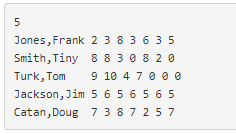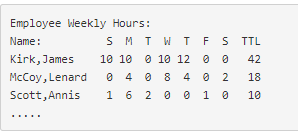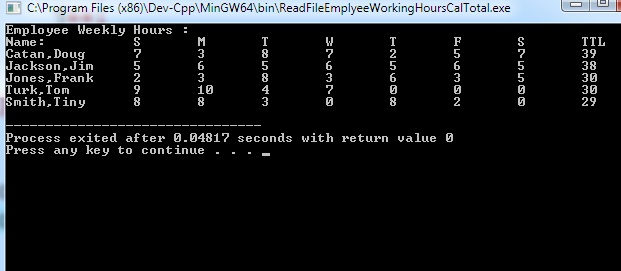# Question & Answer: Write a C++ program that will need to read from a file of employee data for how many hours each employee worked…..

Write a C++ program that will need to read from a file of employee data for how many hours each employee worked for a week. Then for each of the employees, a total of the hours worked is made and then the employees are sorted into descending order based on their total hours using bubble sort.

Input file looks like this:

Don't use plagiarized sources. Get Your Custom Essay on
Question & Answer: Write a C++ program that will need to read from a file of employee data for how many hours each employee worked…..
GET AN ESSAY WRITTEN FOR YOU FROM AS LOW AS \$13/PAGEOutput should look something similar to this:Jones,Frank 2 3 8 3635 Smith, Tiny 883082 Turk, Tom 9104700 e Jackson,Jim 5 6 56 5 6 5 Catan,Doug 7 387257

workedHours.txt

5
Jones,Frank 2 3 8 3 6 3 5
Smith,Tiny 8 8 3 0 8 2 0
Turk,Tom 9 10 4 7 0 0 0
Jackson,Jim 5 6 5 6 5 6 5
Catan,Doug 7 3 8 7 2 5 7

________________

#include <fstream>
#include <iostream>
using namespace std;

void bubbleSort(int totWH[], string names[], int workedHrs[], int size);

int main()
{
// Declaring variables
int size;
string name;
int hours;
int tot = 0;
int i = 0, j = 0, k = 0;

// defines an input stream for the data file
ifstream dataIn;

// Opening the input file
dataIn.open(“workedHours.txt”);

if (dataIn.fail())
{
return 1;
}
else
{
// Reading the data from the file
dataIn >> size;

// Creating arrays
string names[size];
int totWH[size];
int workedHrs[size];

/* reading the data from the file
* and populate the data into arrays
*/
while (dataIn >> name)
{
names[i] = name;

while (dataIn >> hours)
{
workedHrs[j][k] = hours;

tot += hours;
k++;
}
totWH[i] = tot;
tot = 0;
i++;
j++;
k = 0;
dataIn.clear();
}

bubbleSort(totWH, names, workedHrs, size);

// Displaying the data
cout << “Employee Weekly Hours :” << endl;
cout << “Name:ttStMtTtWtTtFtStTTL” << endl;

for (int i = 0; i < size; i++)
{
cout << names[i] << “t”;
for (int j = 0; j < 7; j++)
{
cout << workedHrs[i][j] << “t”;
}
cout << totWH[i] << endl;
}

// Closing the intput file
dataIn.close();
}

return 0;
}
void bubbleSort(int totWH[], string names[], int workedHrs[], int size)
{
int i = 0;
int j = 0;
int temp, temphr;
string tname;

// Performing the sorting(decending order) using bubble sort
for (int i = 0; i < size; i++)
{
for (int j = 1; j < (size – i); j++)
{

if (totWH[j – 1] < totWH[j])
{

temp = totWH[j – 1];
totWH[j – 1] = totWH[j];
totWH[j] = temp;

tname = names[j – 1];
names[j – 1] = names[j];
names[j] = tname;

for (int h = 0; h < 7; h++)
{
temphr = workedHrs[j – 1][h];
workedHrs[j – 1][h] = workedHrs[j][h];
workedHrs[j][h] = temphr;
}
}
}
}
}

_________________________

Output: